# leetcode *990. 等式方程的可满足性（并查集题目表）（2020.6.8）Leetcode 同时被 2 个专栏收录529 篇文章 2 订阅

### 【题目】*990. 等式方程的可满足性

输入：["c==c","b==d","x!=z"]



1 <= equations.length <= 500
equations[i].length == 4
equations[i] 和 equations[i] 是小写字母
equations[i] 要么是 ‘=’，要么是 ‘!’
equations[i] 是 ‘=’

### 【解题思路1】并查集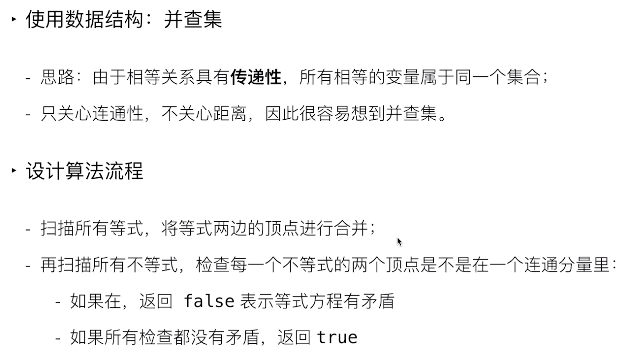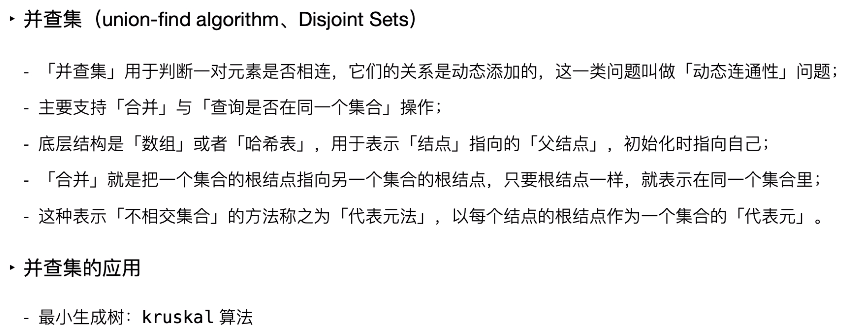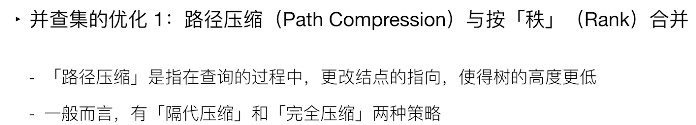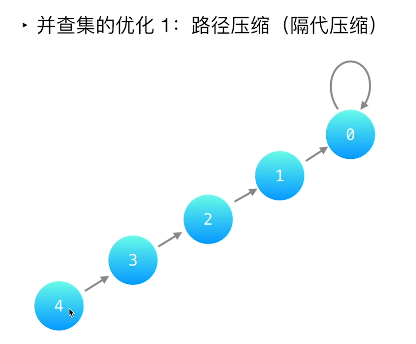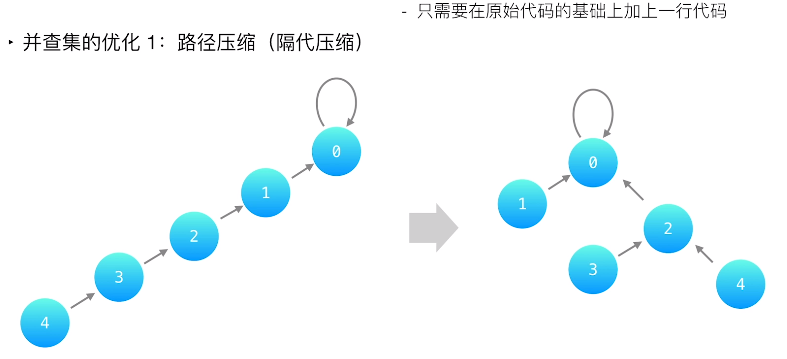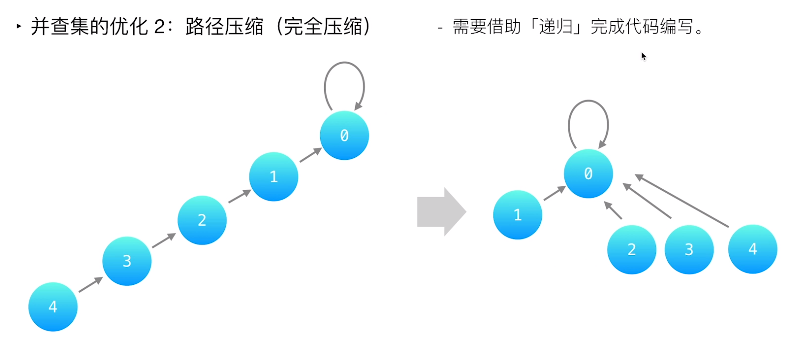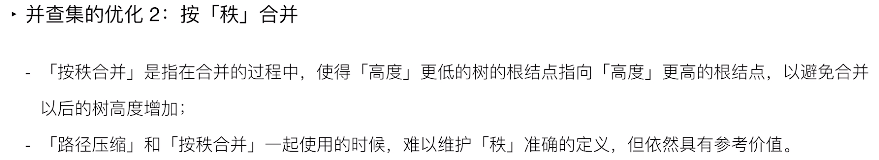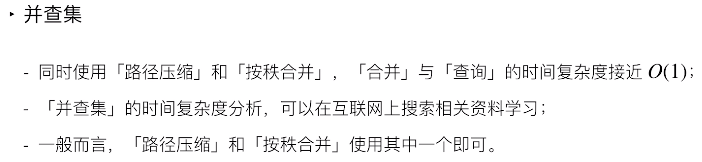class Solution {
public boolean equationsPossible(String[] equations) {
int length = equations.length;
int[] parent = new int;
for (int i = 0; i < 26; i++) {
parent[i] = i; //初始化每个结点是一个单独的集合
}
for (String str : equations) {
if (str.charAt(1) == '=') {
int index1 = str.charAt(0) - 'a';
int index2 = str.charAt(3) - 'a';
union(parent, index1, index2); //将等式两端结点放到同一个连通分量里
}
}
for (String str : equations) {
if (str.charAt(1) == '!') {
int index1 = str.charAt(0) - 'a';
int index2 = str.charAt(3) - 'a';
//合并失败，表示有矛盾，不等式的两个结点在同一个连通分量里
if (find(parent, index1) == find(parent, index2)) {
return false;
}
}
}
//检查完所有不等式，每个不等式的两个结点都不在同一个连通分量里
return true;
}

public void union(int[] parent, int index1, int index2) {
//把index1的根结点挂到index2的根结点上
parent[find(parent, index1)] = find(parent, index2);
}

public int find(int[] parent, int index) {
//根结点才指向自己，即parent[x] == x才说明找到了根结点
while (parent[index] != index) {
parent[index] = parent[parent[index]]; //路径压缩
index = parent[index]; //往上找根结点
}
return index; //返回根结点
}
}


### 并查集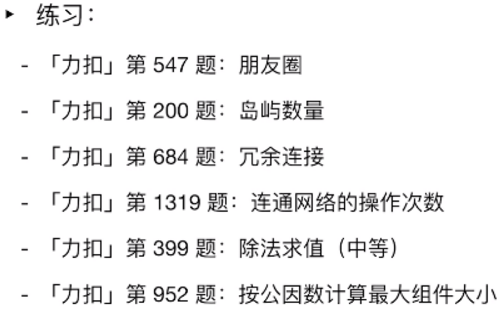点击重新获取扫码支付余额充值